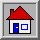# Logic  the Art of Reasoning

Mathematics   the Art of Studying Patterns Using Logic

# The Number Chain### Editorial Note

This document is a work in progress. At this time it contains only the introduction to the number chain and very few activities. Additional information and activities can be found in other Teaching documents of MathVentures.

#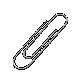Why a Chain?

The number line is an extremely intuitive means to describe the numbers, from the counting numbers (the integers), through the measuring numbers (the fractions) and all the way to the estimating numbers (irrational) [see Figure 1.] With the number line it is simple to describe and easy to see the relationships between numbers, how to construct various numbers from others and so forth. As we will see in an example, the number is useful for demonstrating how fractions are derived from the whole numbers.

The number line suffers from one important drawback. The number-sense topics which we teach with the number line target very young students, grade K through three. Yet many students in these grades have yet to develop the fine-motor skills to draw lines and other objects. So, if we base the teaching of the number line solely on drawings, many students will not be able to participate in hands-on learning.

We have introduced the number chain to overcome this drawback.

The number chain is a simple tool with which you can teach all of the numbers you will ever have to teach in grades K12, the properties of the numbers, the four basic operations and many other relationships. You can easily construct a number chain from readily available, inexpensive material, such as paperclips.#The Number Line

The number line is an intuitive way to represent all the numbers (except for the imaginary numbers, which, as the name indicates, are not quite intuitive.) Further, using the number line, it is possible to teach, learn and develop deep comprehension of the four basic arithmetic operations and of many properties of the numbers, and the relationships among them. For example, using the geometric properties of the number line we can generate as many counting numbers as we need or desire, thereby demonstrating the infinity property of the counting numbers. Multiple instances of the number lines can be combined to create the planar, XY coordinate system and the spatial XYZ coordinate system.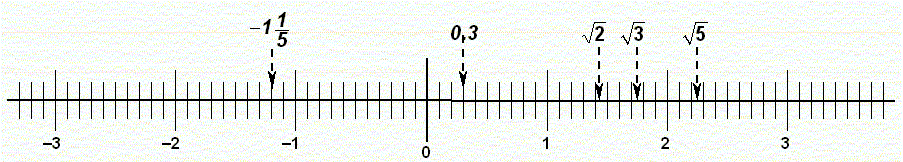Figure 1.    The Number Line#The Number Chain  a Hands-on Model of the Number Line

Constructed from identical paper clips, the number chain is so intuitive that even very young students do not need special instructions how to construct their own. At most, a teacher may tell the students to make sure that all of the paper clips are linked on the outer loop and all are oriented in the same direction.

Figure 2.    The Number Chain, Showing Only the Counting Numbers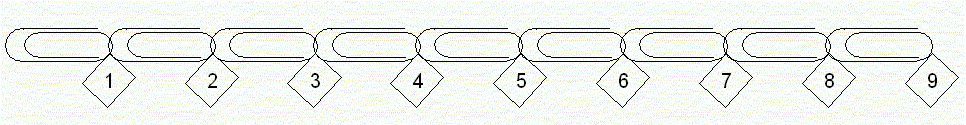Once the students have a paper-clip chain, they need to give each paper clip its numerical name. The students can do it quite easily by simply counting the clips. The question of in which direction to perform the counting is usually avoided if the chain is oriented horizontally on the tabletop in front of the student because our lingual custom to read and write from left to right. However, if it does come up, suggest to the student to count starting at the left-most end of the chain for that very reason.

The next and last element of the number chain is the labels. Once students become familiar with the number chain, they no longer need labels to use and work with it. However, at the early stage, labeling each paper clip with its numerical name deepens the comprehension of counting and numbers.

The mechanic of labeling. One simple way to affix the labels is to use short trims of masking tape. The students do the following:

1.    Tear off a piece of tape;

2.    Write on it the desired number;

3.    Place the labeled tape over the paper clip, sticking it to the tabletop.

Note that, in order to teach the fundamentals of numbers and the basic arithmetic operations, the chain does not have to form a straight line. In fact, logistically it is advantages to let the chain curve on the tabletop or on the floor to conserve workspace. (This is a common practice for Montessori students while working with the bead chains.)

The question to pose to the students is where should we place each label on its corresponding paper clip?

There are three alternatives:

• At the beginning of the clip (its left end.)
• Somewhere in the middle.
• At the end of the clip (its right end.)
Often, most students place the labels at the middle of whichever objects the count; paper clips are no exception. Some students may place their labels at the end while very few, if any, will do so at the beginning.

You settle this issue by saying something like, we place each label after we finish to count each paper clip, while simultaneously demonstrating counting and labeling paper clips, one at a time. (See Figure 2.)

The significance of labeling the paper clips that represent numbers by the end tip of each clip will become even more apparent when we introduce the measuring numbers (fractions) with the number chain.

This first number chain, as seen in Figure 2, represents the counting numbers. In general, each paper clip represents the unit 1 and on the chain each paper clip is the number which is on its label (the label attached to its right end.)

• The opposite or counting backward numbers are formally known as the natural numbers or positive integers.
•##Number Chains from Snap-Cubes and Other Manipulatives

Any type of identically sized, interlocking objects can be used to generate a number chain. Such objects are various type of snap cubes. Using such cubes has the some advantages over using paper clips: The cubes do not get tangled and easily form and maintain a straight line. Also having cubes of different colors will come in handy while teaching some lessons. On the other hand, snap cubes have several disadvantages: They are more costly than paper clips, having various colors may be confusing, and the cubes can be arranged to form two and three dimensional objects. While the later property is a disadvantage for lessons dealing with linear chain, it will become very useful during advanced lessons.

It is important to recognize that different students have different fine-motor and dexterity skills. Some can manipulate paper clips better and others are more adapt working with snap cubes.

There are other supplies that can be used to create a number chain. For example, there are various types of large and colorful plastic links that are often used to create chains for infants. Chains created from such links are very effective demonstration aids. But students who lack dexterity to work with paper clips are often able to manipulate and work with these much larger plastic links.

For these reasons we often start working with paper clips but use snap cubes whenever they are more suitable.#Addition and Subtraction

Using the basic number chain students can perform several operations.
• Counting. Using their individual chains, students count
• Individual paper clips or cubes;
• Pairs, triplets and other groups of multiple objects.
• Addition. To their current chains, students link additional chain. The total length of the combined chains can be determined by counting or measuring relative to a long, reference chain.
• Subtraction. From their current chains, students remove a specified number of links. The remaining length of the chains can be determined by counting or measuring relative to a long, reference chain.
Of course, these are only the most elementary activities. You are free to devise as elaborate activities as your students can handle so they master the skills.#The Counting-in-Place Number

 Lets look at the labels of the numbers on the chain again and ask: Shall we label the leftmost end of the chain? and If so, what number shall we write on this leftmost label?  The answers are yes and 0 respectively. We can look at the number zero as the counting in place number. A simple activity demonstrate the counting-in-place property of zero: Instruct a student to use his or her steps to measure the classroom width or length. As the student advances from one side of the classroom toward the other, he or she encounters obstacles, which he or she must step around. How should the counting proceed while stepping around the obstacles?  For each side step, the student should repeat the last number counting advancing toward the target. Figure 3 shows an example. Figure 3.    Measuring Distance by Footsteps, notice the use of the Counting in Place Number.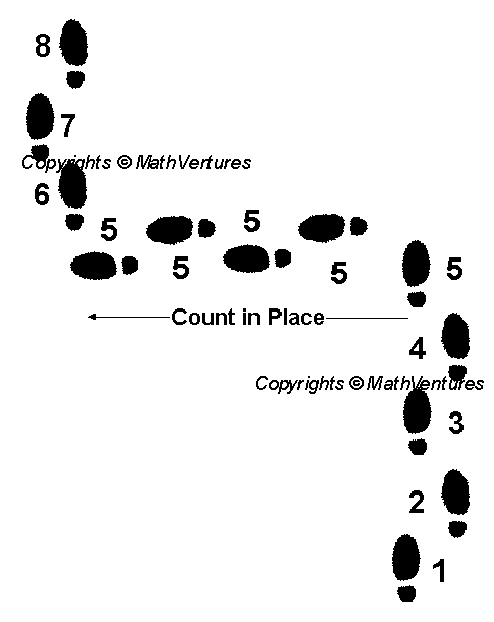Zero is a very important number. Not only we use it when counting in place but it is also the name of the number before we start counting. That is the reason for placing a label marked 0 at the leftmost end of the chain.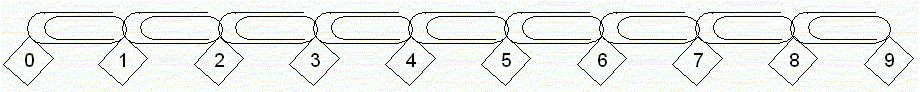Figure 4.    The Number Chain with the Zero Marker#The Opposite Numbers

Now instruct your students to add links to the chain starting at the zero point and advancing leftward. There is nothing different about these paper clips. There is nothing different about these paper clips that distinguishes them from the ordinary counting numbers. These are opposite numbers. It is nothing more than an arbitrary custom  formally we say a convention  that is very convenient, useful and helpful.

Figure 5.    The Number Chain with All Counting Numbers.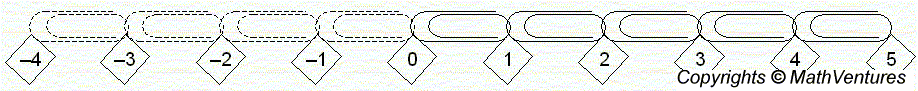We can now add more activities. The opposite numbers can be used as counting backward or counting down numbers. It is important to point to the students that we use the words backward and down in relative terms. That is, whichever direction we opt to designate as forward or up, the opposite is backward or down. This can be right versus left, up versus down, forward or backward. For example, the ground level of an elevator that is going down into a mineshaft may be zero, then the next level down is 1, the next 2 and so forth. In this case, going downward the levels are numbered with positive numbers. The same is true for divers who count the depth of their dives.

No we see that zero is not only the counting in place number. On the number chain it separates the counting numbers from the opposite numbers  Since it is found at the very beginning of both groups of numbers we also call it the origin.#All Counting Numbers

• The opposite or counting backward numbers are formally known as the negative numbers.
• Together with zero and the opposite numbers the number chain now represents all counting integers.
• All the counting numbers are formally called the integers.

##Counting, Adding and Subtracting

Using the expanded number chain students can perform several operations.
• Counting. Using their individual chains, students count
• Individual links forward and backward
• Measure distances, in units of links, between objects, having obstacle between them
• Pairs, triplets and other groups of multiple objects.
• Subtraction. Subtracting both counting and opposite numbers.
Again, these are only the most elementary activities. You are free to devise as elaborate activities as your students can handle so they master the skills.Continue reading: Part 2, Advanced Subjects